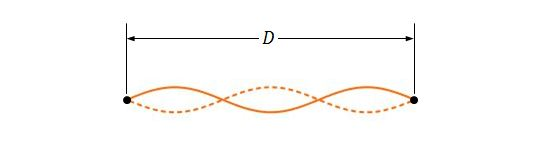Classical Mechanics

# Boundary conditions of standing waves

If two adjacent resonant frequencies are $$440$$ and $$330\text{ Hz}$$ for a string which is stretched to a length of $$77.0\text{ cm},$$ what is the lowest resonant frequency?

Consider a wire with length $$8.0\text{ m}$$ and mass $$110\text{ g}$$ which is under a tension of $$290\text{ N}.$$ What are the lowest and the third lowest frequency for a standing wave on the wire?

Consider two identical sinusoidal waves with the same wavelength and amplitude traveling in opposite directions along a string with a speed of $$30.0 \text{ cm/s}.$$ If the time interval between adjacent instants when the string is flat is $$0.100\text{ s},$$ what is the wavelength of the waves?

If a string with length $$5.50\text{ m},$$ mass $$0.120\text{ kg},$$ and tension $$98.0\text{ N}$$ is oscillating, approximately what are the longest possible wavelength and frequency for a standing wave?Suppose that a string, two ends of which is fixed at a distance of $$D=120.0\text{ cm},$$ has a linear density of $$7.4\text{ g/m}$$ and is under a tension of $$150\text{ N}.$$ If the string is oscillating in a standing wave pattern, as shown in the above figure, what is the approximate frequency of the traveling waves?

×